# CIFAR-10

CIFAR-10 computer-vision training dataset

The CIFAR-10 dataset contains 50,000 training and 10,000 test images of 10 object classes ("airplane", "automobile", "bird", "cat", "deer", "dog", "frog", "horse", "ship", "truck"). Each image is an RGB image of size 32x32.

## Examples

### Basic Examples

Retrieve the resource:

 In:=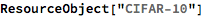Out=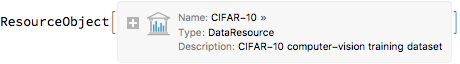View the data:

 In:=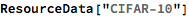Out=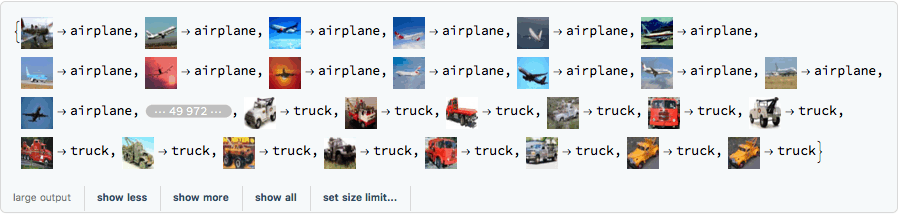### Analysis

Obtain a random sample of training data:

 In:=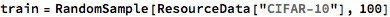Out=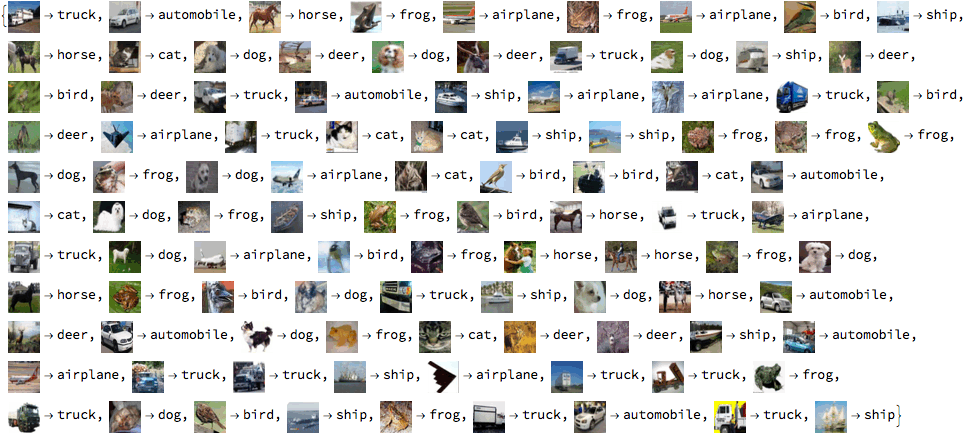Train a classifier:

 In:=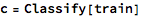Out=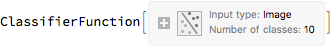Obtain general information about the classifier:

 In:=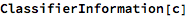Out=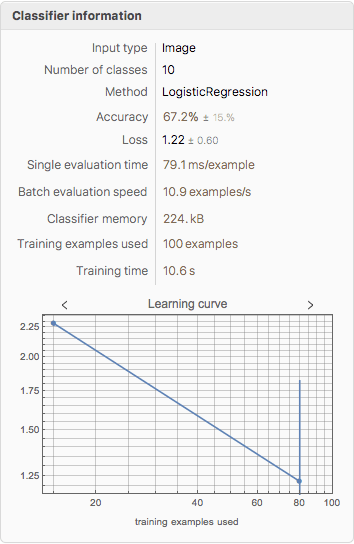Obtain a random sample of test data:

 In:=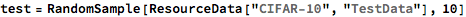Out=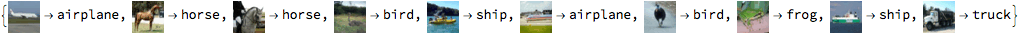Generate a ClassifierMeasurementsObject of the classifier with the test set:

 In:=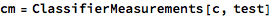Out=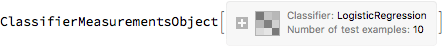Visualize the accuracy of the classifier:

 In:=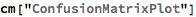Out=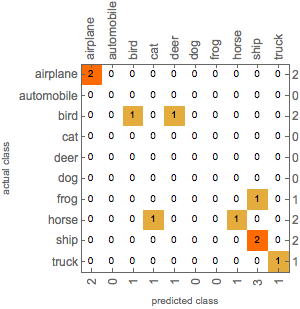Wolfram Research, "CIFAR-10" from the Wolfram Data Repository (2018) https://doi.org/10.24097/wolfram.83212.data